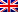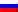# Maximum flow problem

Contenus

## Maximum flow

The maximum flow to model a very large class of problems. Their interpretation corresponds to the circulation of physical flows on a network: electrical distribution, adduction network, routing of packets on the Internet, etc. This involves conveying the greatest possible quantity of material between a source s and a destination t.

## Definition of a network

A network is a directed graph N = (V, A) with a positive valuation of its edges. The valuation c (x, y) of an arc (x, y) is called the capacity of the arc. N has two particular vertices: a source s and a destination t. The other vertices are called intermediate nodes.

A flow represents the routing of a flow of materials from a source s to a destination t. The flow is thus described by the quantity of material passing through each of the arcs of the network. This quantity must be less than the capacity of the arc, which thus limits the flow that can pass through it. In addition, it is not possible to store or produce matter at the intermediate nodes: a flow locally verifies a conservation law similar to Kirchhoff's laws in electricity.

A flow F on a network N is a positive valuation of the arcs which satisfies the following two properties:

1. For any bow a ∈ TO, 0 ≤ F (a)that)
2. For any intermediate summit xV \ { s, t }, ∑y F (y, x) = ∑y F (x, y)

The sum F(x) =yF (y, x) is the inflow at the top x
The sum F+(x) =yF (x, y) is the outgoing flow Summit x
The value | F | of a flood F is defined as the outgoing flow minus the incoming flow in s : | F | = F+(s)F(s).

## Maximum flow problem

The classical maximum flow problem is a linear problem. We assume that the network has edges between any pair of vertices. If there is no capacity, it is fixed at 0. The objective function is the sum of the flows leaving the source or entering the sink. The lens function may vary depending on the lens.

The basic constraints are identical whatever the objective function:

• Capacity constraints: f (u, v) ≤ c (u, v)
• Symmetry: f (u, v) = - f (v, u)
• Conservation of flows: the sum of the incoming flows is equal to the sum of the outgoing flows except for the source and the sink, the degree d (u) is called the difference between the outgoing and incoming flow from the vertex u: d (u) = 0 except for u = s and u = t.
• others

Many problems can be traced back to a maximum flow problem. A naive algorithm is to repeat the following process until you get stuck. Find a path st where each arc has (e) <u(e) et augmenter le débit le long du chemin par le minimum de u(e)-f(e). Cette méthode ne donne pas une valeur optimale, nous devons être en mesure de revenir en arrière.

## Reduction of problems

Multi-source multi-well flow problem : a fictitious source is connected to all the sources, the capacity of the edges depends on various criteria (capacity of the vertices, inflow-outflow constraints). The wells are connected to a fictitious well. This type of problem is usually a pairing problem.

Flow problem with capacity at the peaks : the vertices are duplicated in the entering vertex and the outgoing vertex with an arc connecting them. The capacity of this arc is equal to the capacity of the top.

Shortest path problem : the source is the origin of the path and the sink with d (s) = 1 and d (t) = - 1. There are no capacity constraints. The cost of passage in all arcs is 1. We are looking for the minimum cost stream.

## Network cut and residual capacity

A cut (E, T) of a transport network N = (V, A) is a partition of V into E and T = VE such that s ∈E and t∈T. We define the capacitance c (E, T) of the cross-section the sum of the capacitances of the arcs (u, v) with u in E and v in T. For any cross-section (E, T) and any flow f, | f | is increased by the capacity of the cut c (E, T).

Remove a set of edges to disconnect t from s. Find a set to minimize the sum of the capacities of the arcs. A min cut is a partition of nodes (S, T) such that s is in S and t in T where c (E, T) is minimal. By definition, the min-cut problem has the same result as a maximum flow problem.

Given a network N = (V, A) and a flow f over N, we call residual capacity cf (u, v) = c (u, v) - f (u, v). Moreover, if the capacity of (v, u) is zero, cf (v, u) = f (u, v). The residual capacity is always positive or zero.

The residual graph is the network N '= (V, A) with the residual capacities for each arc of A. An increasing path is a path between s and t in the residual graph.

From the residual graph of a max flow, it is possible to find the solution of the min-cut problem (and vice versa). In the following graph, if you find a set of connected vertices from the vertex s, you find the set {s, 3,4,7} which is the set S for the min-cut problem.

## Find an increasing flow

Find a path st in the residual graph, it is called an increasing path. Once the path is selected, increase the flow along the arcs in the same direction as the standard graph, decrease the flow along the backward arcs.FRFRENRUES